# 2 Air interface

## 2 Air interface

The purpose of the air interface is to adapt the signal to the transmission channel and to optimise the quantity of information transmitted from the source to the receiver.

At the transmit side it consists of wave shaping functions with forward error correction often with interleaving, modulation, radiofrequency devices (filter, amplifier , antenna, frequency converter etc) and multiple access techniques for the simultaneous transmission of several signals in the same transmission channel. (Figure 11.1).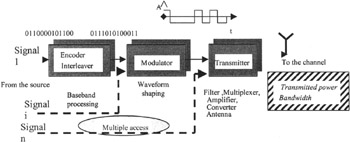Figure 11.1: Air interface, transmission

At the receive side after antenna and amplification and frequency conversion, are implemented demodulation, and specific techniques, e.g. diversity, for correction of the channel distortions (Figure 11.2).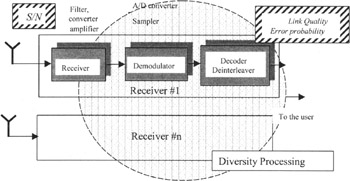Figure 11.2: Air interface, reception

### 2.1 Transmission channel

Adapting the signal to the channel assumes a good knowledge of the propagation mechanisms. They are defined by the laws of electromagnetism and physical characteristics of the propagation media.

#### 2.1.1 Characteristics of smart device links

1. Distances and environment
Links between smart devices may exist in different environments:

• cellular networks, with distances ranging from several hectometres to several kilometres in urban and suburban environments with many obstacles,

• Radio LAN for indoor coverage and also outdoor picocells (campus) with distances of a few tens of meters ,

• domestic or personal networks with distance of a few meters to connect terminal often but not always in line-of-sight conditions.

2. Frequency bands
A very wide spectrum, depending on radio regulations, is possible for smart device links from some hundreds of MHz to a few tens of GHz and beyond in the infrared and optical bands. The low limit is due to the size of antennas and the high limit to coverage problem considering the possible transmitted power. At present, the frequency bands most used are 806 “960 MHz (GSM, AMPS), 1700 “1900 MHz (GSM, DECT, PCS), 1920 “1980/2110 “2170 and 1900 “1920/2010 “2025 MHz (UMTS FDD and TDD), 2,4 “2,5 GHz and 5 GHz for RLAN, 3,5 and 26 and 40 GHz (40.5 “43.5 GHz) for wideband fixed access. The 54.2 “66 GHz band is considered for mobile broadband indoor access (MBS). Infrared networks also exist.

3. Bandwidth occupancy
The trend is an increase of bit rates for most of the multimedia services. Bit rates of tens of Mbit/s are envisaged for video access. Generally , the larger the mobility is, the lower are the bit rates (see Figure 11.3). A macrocellular network will not provide Mbit/s to high velocity mobiles. The bandwidth occupancy is directly related to the bit rate and coding and modulation parameters.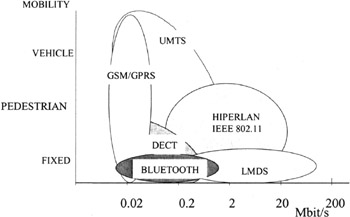Figure 11.3: Mobility and bit rate

#### 2.1.2 Propagation effects

Path loss is the first effect of propagation. Free space loss expressed in decibel increases as 20 log d where d is the distance and as 20 log f where f is the frequency. Using higher frequency bands is therefore costly because the possibility to trade off the propagation loss by directive antennas, easier to implement at higher frequency, is not really possible in a mobile context.

In a mobile cellular environment, propagation is rarely in a line-of-sight (LOS) situation but more often in non-line-of-sight (NLOS) depending on reflection and diffraction. Roughly , the loss is 35 log d. The NLOS propagation is limited to lower frequencies up to a few GHz because the diffraction losses, increasing as 10 log F, become too large. So it is sensible to use the lower frequencies for cellular networks and higher frequencies for short range communications. On the contrary, reflections still exist at high frequencies. Globally, in most of the cases multipath propagation occurs, created by the addition of several replicas of the transmitted signal with different amplitudes and delays. The multipaths can be classified into two categories; small scale multipaths or rapid fadings due to obstacles very close to the receiver (somme wavelengths ) which are modelled by Rayleigh (NLOS) and Rice (LOS) processes; and large scale multipaths corresponding to reflection and diffraction on distant obstacles, expressed by the impulse response or the power delay profile of the channel. The delay spread D r second order moment of the power delay profile or coherence bandwidth B c ( satisfying DrBc > constant) are frequently used to characterise these selective fadings. The range of Dr is large depending on the frequency band and the environment, from a few ns in a room at 60 GHz to several tens of ¼ s in mountainous terrain. Channel impulse response models are recommended especially for the comparison of 3G air interfaces and it is important that realistic models are available to estimate the performances .

A rule of thumb is to compare the symbol rate Ds to Dr (or the signal bandwidth to the coherence bandwidth). If Dr > Ds (or Bs > Bc) then the channel will distort the signal.

#### 2.1.3 Other channel distortions

The transmission channel in a wide acceptance including transmit and receive radio frequency equipment (antennas, amplifiers , frequency converters) is characterised by different kinds of noise, sky noise, thermal noise or low noise amplifier, interferences caused by other signals (co-channel interference in the same frequency band from neighbouring cells , adjacent channel interferences from adjacent frequency bands). In the simplest approach, the noise is modelled as a white additive Gaussian of constant density No/2.

### 2.2 Performance of radio interface

The main criteria to evaluate the performances of the air interface are:

1. spectrum efficiency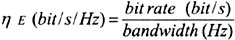or more generally for cellular systems with frequency reuse in various cells the cellular spectral efficiency2. link quality : essentially error probability (bit error rate or frame error rate), and others variables like delay conditions for decoding: some services such as voice services being strongly delay-constrained contrary to data services which accommodate several hundreds of ms.

3. signal to noise ratio of the signal power S divided by the noise power N (usually in digital communications a ratio Es/No or Eb/No symbol energy or bit energy to noise density).

Note that these criteria could be inconsistent, i.e. a better spectral efficiency could be obtained with a higher interference level at the expense of the BER. Optimisation choices will depend on the system itself.

#### 2.2.1 Modulation and coding

The maximum bit rate (or capacity per time unit) for which the error probability can be reduced arbitrarily small in a white additive Gaussian noise channel of bandwidth B is given by the famous Shannon formula: DC(bit/s) = B log 2 (1 + S/N ). It imposes a theoretical limit and recent results in coding and modulation have shown that it could be closely approached.

Forward error correction is obtained by introducing N-K redundancy bits computed from K information bits in order to make the codewords ˜more distant in terms of a given distance (often the Hamming distance, number of positions where two codewords differed). The coding rate R is the ratio K/N (R < 1). The code performances depend on the minimum distance and roughly they are improved when for given N and R, the distance increases.

Modulation is defined by the number M and the position of states or points in a constellation, the waveform which determines the power spectral density (important for interferences considerations) of the transmitted signal. For M 8, the classical modulations are PSK (phase-shift-keying) modulations BPSK, QPSK, 8-PSK and for M> 8 QAM (quadrature amplitude modulation). 16-QAM constellation is given in Figure 11.4. In-phase (cos (2 fo + o)) and in-quadrature carrier (sin (2 fo + o)) are the two axes.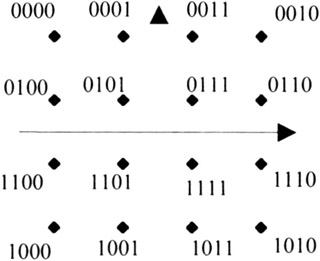Figure 11.4: 16-QAM constellation

Like FEC, performances are related to a distance (often the Euclidean distance). For a given average power, the further the points in the constellation, the lower is the error probability. This implies that the error probability increases as the number of points, i.e. for M-PSK modulation where all the points are equally distributed on a circle with the same radius, the distance between two adjacent points is smaller when the number of points is larger.

BER is generally plotted versus the Eb/No ratio. The shape of these plots is given in Figure 11.5. Practically, BER objectives after FEC decoding on a radio link depend on the type of services in the range of 10 -3 to 10 -10 .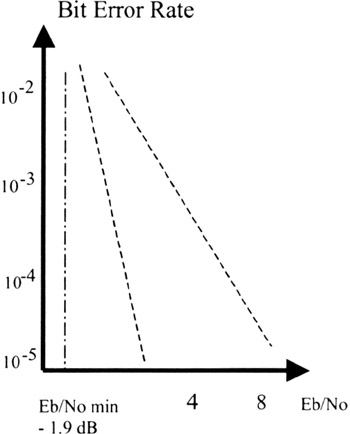Figure 11.5: BER vs. Eb/No

Revisiting the formula giving the maximum bit rate R < C to obtain an arbitrarily small error probability, it can be derived that the theoretical limit for Eb/No is -1.9 dB. At present, the best coding schemes provide BER of 10 -5 for about 1 dB.

For a given bit rate Db, the symbol rate Ds is expressed by Ds = Db/ (R log 2 M). The signal bandwidth B varies as Ds. The spectrum efficiency · E is therefore improved with modulations having a large number of points M and FEC of high coding rate R. For instance, EDGE owing to 8-PSK has a spectrum efficiency roughly 3 times better than the GMSK modulation of GSM, which behaves like a binary modulation.

Combination of modulations with an adjustable number of states and variable rate FEC is one of the key issues of link adaptation which provides the best compromise between link quality and spectrum efficiency in given propagation conditions. One of the most significant example is HIPERLAN2 air interface which combines different codes and modulations enabling, in the same 20 MHz bandwidth, a low bit rate of 6 Mbit/s with R = ½ and M = 2 and a high bit rate of 54 Mbit/s with R = ¾ and M = 64 according to the propagation conditions. (Bertin paper).

#### 2.2.2 Multiple access

Multiple Access Techniques are designed to transmit simultaneously several signals in the same transmission channel. The basic principle is signal orthogonality.

Frequency Division Multiple Access (FDMA) has been used in the first generation of mobile systems. It has been drastically improved with OFDM ( orthogonal frequency division multiplexing ) which gives a better spectrum efficiency by sparing guard bandwidths between carriers .

Time Division Multiple Access (TDMA) is implemented in GSM on a single carrier. Several carriers are frequency multiplexed in the allocated bandwidth. Frequency reuse in a cellular scheme is the key factor for cellular spectrum efficiency.

Code Division Multiple Access (CDMA) is used for IS-95. Every information bit of a signal for a given user is ˜multiplied by a digital sequence (the spreading code) made of ˜chips . This technique, also known as direct sequence spread spectrum , is a spin-off of Shannon's results on the channel capacity. The UMTS air interface is based on an improved version, wideband CDMA (WCDMA). Another spread spectrum technique is frequency hopping . It is specified in the Bluetooth standard. For RLAN, the 802.11b standards are based on CDMA, while 802.11a is an OFDM access. Spectrum efficiency comparisons between all these techniques are usually tricky, depending on a lot of parameters.

Multiple access techniques have also specific properties relevant to the channel distortions. For example, OFDM by splitting the signal on several carriers, is robust to selective fadings but sensitive to non linearities. Rake receivers exploit the CDMA spreading properties to combine multipaths and improve BER.

Spatial Division Multiple Access (SDMA) is another type of multiple access based on antenna arrays. Beamforming allows the array to focus the antenna pattern simultaneously towards different mobiles located in distinct spatial directions.

#### 2.2.3 Diversity techniques

1. Time diversity : FEC with interleaving spread the information on several bits in different time slots in order to correct the error bursts due to rapid fadings. Bit interleaved coded modulations (BICM) provide a robust solution with a good spectrum efficiency for channels with and without fading.

2. Frequency diversity : OFDM and CDMA spread the information on several frequencies or frequency bands and offer a better resistance to selective fadings than a single carrier access.

3. Spatial diversity : Using several receive antennas to decorrelate rapid fadings is a well known technique. Antennas arrays could be considered as reconfigurable directive antennas which minimised the effects of multipaths and interference from other users.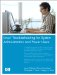Linux Troubleshooting for System Administrators and Power Users
ISBN: N/A
EAN: 2147483647
Year: 2005
Pages: 191

Similar book on Amazon